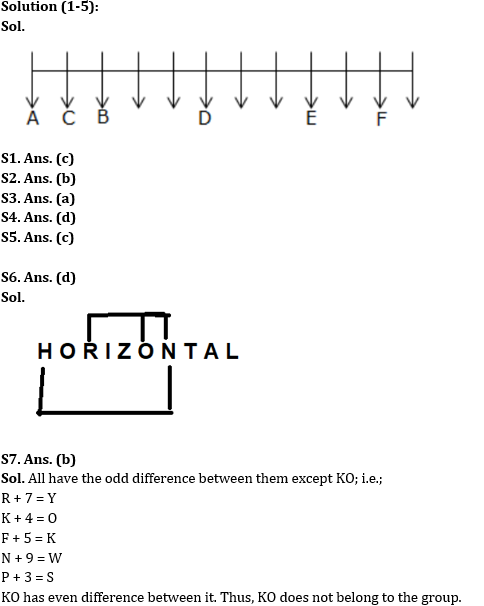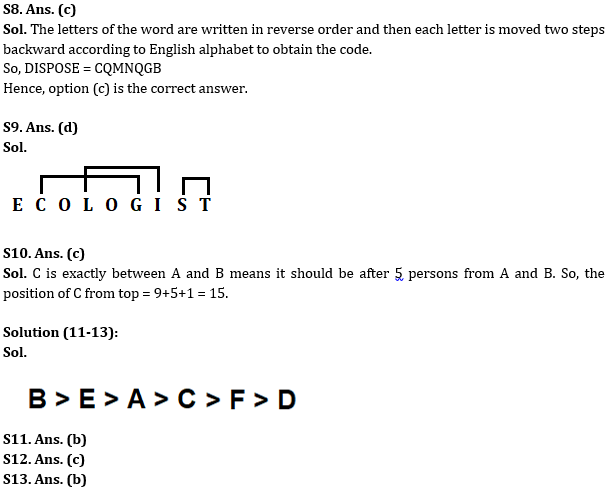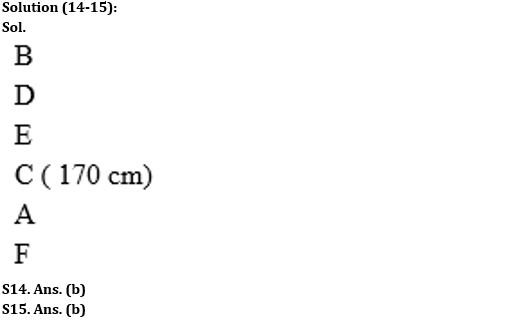Latest Banking jobs   »

# Reasoning Ability Quiz For SBI Clerk Prelims 2022- 18th May

Directions (1-5): Study the following information carefully and answer the given questions.
A certain number of people sit in a linear row and face towards the south direction. Only one person sits to the left of F. E sits 3rd to the left of the one who sits 5th to the right of F. Number of members sit between D and E are same as number of members sit between D and B. D sits 4th to the left of C. C and B are neighbors. A is the neighbor of C. No one sit to the right of A.

Q1. How many persons sit between A and E?
(a) Five
(b) Six
(c) Seven
(d) Eight
(e) Nine

Q2. What is the position of D with respect to B?
(a) 3rd to the right
(b) 3rd to the left
(c) 2nd to the left
(d) 2nd to the right
(e) 4th to the right

Q3. How many such persons sit in the row about whom no information is given?
(a) Six
(b) Seven
(c) Five
(d) Three
(e) Four

Q4. Who amongst the following sits at the extreme end?
(a) The one who sits 3rd to the right of E
(b) The one who sits immediate right of D
(c) The one who sits 2nd to the left of B
(d) The one who sits 5th to the right of D
(e) The one who sits 7th to the right of E

Q5. Who among the following sits 7th to the right of the one who sits 3rd to the left of D?
(a) A
(b) B
(c) C
(d) E
(e) F

Q6. How many such pairs of letters are there in the word ‘HORIZONTAL’ each of which has as many letters between them in the word as in the English alphabet (Both forward and backward direction)?
(a) Five
(b) Six
(c) Three
(d) Four
(e) None of these

Q7. Four of the following five are alike in a certain way based on their positions in the English alphabetical series and hence they form a group. Which one of the following does not belong to that group?
(a) RY
(b) KO
(c) FK
(d) NW
(e) PS

Q8. In a certain code, COMFORT is written as RPMDKMA. How would DISPOSE be written in that code?
(a) QGBMNOQ
(b) NMQBCDB
(c) CQMNQGB
(d) CVBQDMN
(e) None of these

Q9. How many are such pairs of letters there in the word ‘ECOLOGIST’, each of which has as many letters between them in the word (in both forward and backward directions) as they have between them in the English alphabetical series?
(a) None
(b) One
(c) Two
(d) Three
(e) More than three

Q10. In a row of 31 people, A is 9th from the top and B is 11th from the bottom. C is equidistant from both A and B. What is the position of C from the top?
(a) 14
(b) 16
(c) 15
(d) 18
(e) None of these

Directions (11-13): Study the information given below and answer the questions based on it.
Six persons A, B, C, D, E, and F are having different weights. C is heavier than F. E is only lighter than one person. A is heavier than D. F is heavier than D. B is heavier than A. The number of people heavier than A is the same as lighter than C. A is heavier than C.

Q11. Who among the following is the heaviest person?
(a) F
(b) B
(c) D
(d) C
(e) None of these

Q12. Who among the following is the lightest person?
(a) A
(b) B
(c) D
(d) C
(e) None of these

Q13. How many persons are lighter than A?
(a) One
(b) Three
(c) Two
(d) More than three
(e) None of these

Directions (14-15): Study the information given below and answer the questions based on it.
Six persons A, B, C, D, E and F are standing in a straight line according to their height. No two persons are of the same height. C is 170 cm tall. D is not the tallest person. F is shorter than A who is shorter than C and D. Only two persons are taller than E, but neither of them is C.

Q14. If A is 163 cm tall, then what could be the height of E?
(a) 160cm
(b) 171 cm
(c) 169 cm
(d) 168 cm
(e) 165 cm

Q15. If all the persons are rearranged alphabetically from left to right, then who will retain his previous position?
(a) E
(b) F
(c) B
(d) A
(e) C

Solutions#### Congratulations!